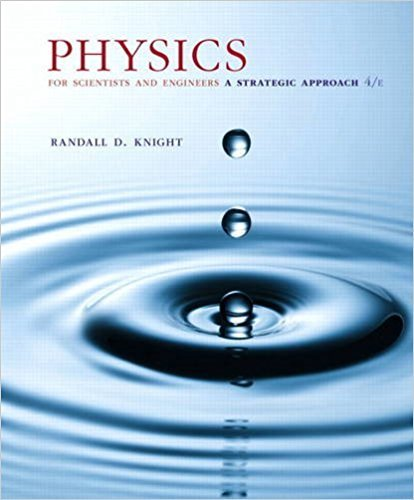×
×

# a. What is the probability that an electron will tunnelISBN: 9780134081496 191

## Solution for problem 40.80 Chapter 40

Physics for Scientists and Engineers: A Strategic Approach, Standard Edition (Chs 1-36) | 4th Edition

• Textbook Solutions
• 2901 Step-by-step solutions solved by professors and subject experts
• Get 24/7 help from StudySoup virtual teaching assistantsPhysics for Scientists and Engineers: A Strategic Approach, Standard Edition (Chs 1-36) | 4th Edition

4 5 0 419 Reviews
21
4
Problem 40.80

a. What is the probability that an electron will tunnel through a 0.50 nm air gap from a metal to a STM probe if the work function is 4.0 eV? b. The probe passes over an atom that is 0.050 nm tall. By what factor does the tunneling current increase? c. If a 10% current change is reliably detectable, what is the smallest height change the STM can detect?

Step-by-Step Solution:
Step 1 of 3

• SpeedingUpIndex o Somethingpersomethingelse=index o something/somethingelse=speed/time=acceleration • Tofindacceleration…(twoways) 1. DataTableWay Example: t v Δt(t 2t 1) Δv(v...

Step 2 of 3

Step 3 of 3

##### ISBN: 9780134081496

This textbook survival guide was created for the textbook: Physics for Scientists and Engineers: A Strategic Approach, Standard Edition (Chs 1-36), edition: 4. The full step-by-step solution to problem: 40.80 from chapter: 40 was answered by , our top Physics solution expert on 12/28/17, 08:06PM. This full solution covers the following key subjects: . This expansive textbook survival guide covers 42 chapters, and 4463 solutions. The answer to “a. What is the probability that an electron will tunnel through a 0.50 nm air gap from a metal to a STM probe if the work function is 4.0 eV? b. The probe passes over an atom that is 0.050 nm tall. By what factor does the tunneling current increase? c. If a 10% current change is reliably detectable, what is the smallest height change the STM can detect?” is broken down into a number of easy to follow steps, and 69 words. Physics for Scientists and Engineers: A Strategic Approach, Standard Edition (Chs 1-36) was written by and is associated to the ISBN: 9780134081496. Since the solution to 40.80 from 40 chapter was answered, more than 223 students have viewed the full step-by-step answer.

Unlock Textbook Solution